# SAT II Math II : Mean

## Example Questions

### Example Question #1 : Mean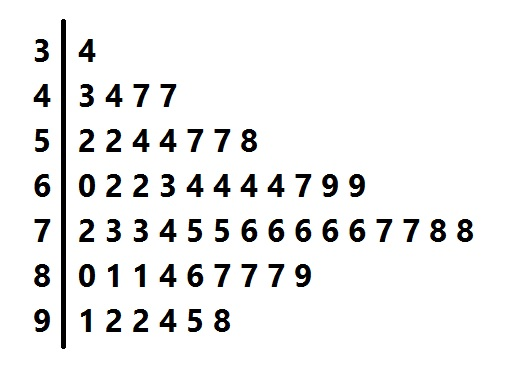Above is the stem-and-leaf display for a group of test scores. Order the mean, the median, and the mode of the scores from least to greatest.

Mode, median, mean

Mean, mode, median

Median, mean, mode

Median, mode, mean

Mean, median, mode

Mean, median, mode

Explanation:

The scores represented can be found from matching the tens digits in the "stem" to the units digits that form the "leaves" in their row. For example, the "leaves" in the "5" row are "2 2 4 4 7 7 8", so the scores will be 52, 52, 54, 54, 57, 57, and 58.

There are 53 scores represented, so to find the median, look for the middle score, in position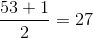.

As can be seen in this diagram, the score - the median - is 74.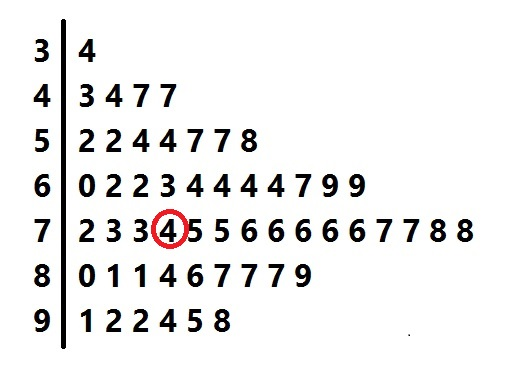The most frequently occurring "leaf" is the "6" in the "7" row, so the mode is 76.

The mean is the sum of the scores divided by 53. If we add the scores, we get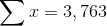, the mean is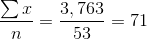In ascending order, the values are mean, median, mode.

### Example Question #2 : Mean

Find the mean of the following data set: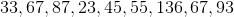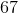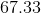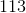Explanation:

Find the mean of the following data set:To find the mean, add up all the terms and divide by the total number of terms.

We have 9 terms, so our denominator will be 9.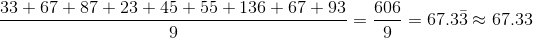So our mean is 67.33

### Example Question #3 : Mean

Find the mode of the data set.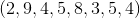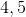Explanation:

The mode of a data set is the data point(s) that appear the most often.

In the data set for this problem, both 4 and 5 appear twice, and no other number appears more than twice.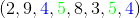So for this data set there are two modes, 4 and 5.

### Example Question #4 : Mean

Find the mean of the following numbers: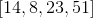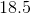Explanation:

The mean is the average of all the number in the data set.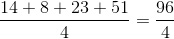The answer is:### Example Question #5 : Mean

Find the mean of the numbers: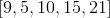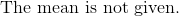Explanation:

The mean is the average of all the numbers given.

Add all the numbers and divide the total by five.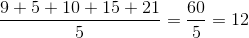The answer is:### Example Question #6 : Mean

Determine the mean: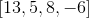Explanation:

The mean is the average of all numbers in the data set.

Add the numbers, and divide the total sum by four, since there are four numbers.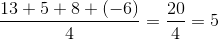The answer is:### Example Question #7 : Mean

Determine the mean of the three numbers: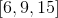Explanation:

The mean is the average of the numbers provided.

Sum the numbers and divide the sum by three.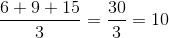The answer is: